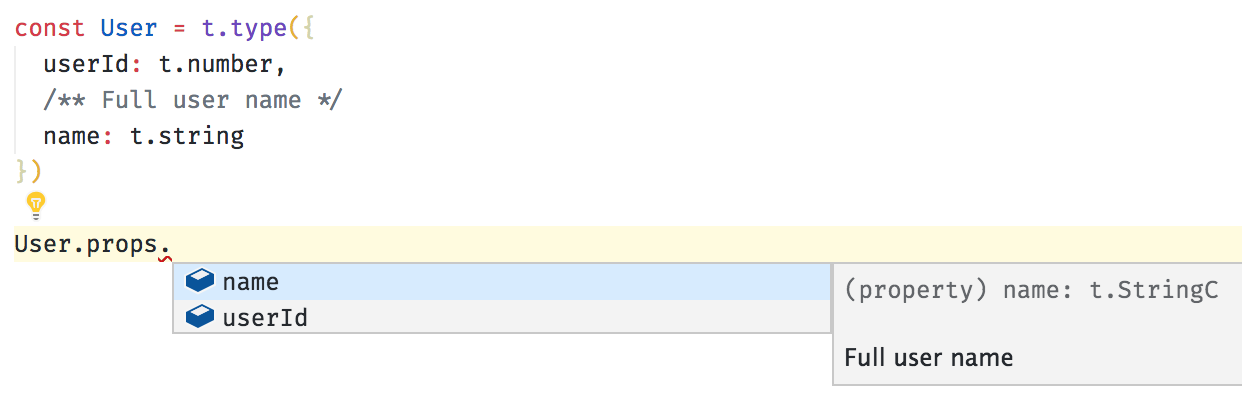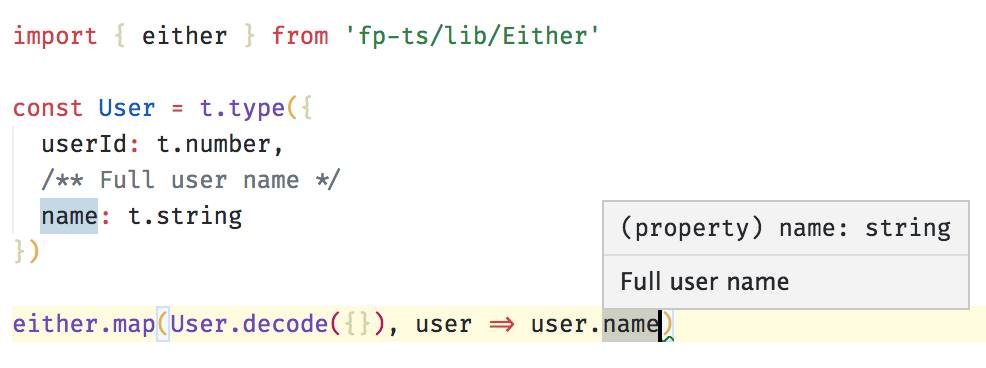# @rlvt/io-ts

TypeScript compatible runtime type system for IO validation

## Usage no npm install needed!

``````<script type="module">
import rlvtIoTs from 'https://cdn.skypack.dev/@rlvt/io-ts';
</script>``````# Installation

To install the stable version:

``````npm i io-ts fp-ts
``````

Note: `fp-ts` is a peer dependency for `io-ts`

# The idea

A value of type `Type<A, O, I>` (called "codec") is the runtime representation of the static type `A`.

A codec can:

• decode inputs of type `I` (through `decode`)
• encode outputs of type `O` (through `encode`)
• be used as a custom type guard (through `is`)
``````class Type<A, O, I> {
constructor(
/** a unique name for this codec */

/** a custom type guard */
readonly is: (u: unknown) => u is A,

/** succeeds if a value of type I can be decoded to a value of type A */
readonly validate: (input: I, context: Context) => Either<Errors, A>,

/** converts a value of type A to a value of type O */
readonly encode: (a: A) => O
) {}

/** a version of `validate` with a default context */
decode(i: I): Either<Errors, A>
}
``````

The `Either` type returned by `decode` is defined in fp-ts, a library containing implementations of common algebraic types in TypeScript.

The `Either` type represents a value of one of two possible types (a disjoint union). An instance of `Either` is either an instance of `Left` or `Right`:

``````type Either<E, A> =
| {
}
| {
}
``````

Convention dictates that `Left` is used for failure and `Right` is used for success.

Example

A codec representing `string` can be defined as:

``````import * as t from 'io-ts'

const string = new t.Type<string, string, unknown>(
'string',
(input: unknown): input is string => typeof input === 'string',
// `t.success` and `t.failure` are helpers used to build `Either` instances
(input, context) => (typeof input === 'string' ? t.success(input) : t.failure(input, context)),
// `A` and `O` are the same, so `encode` is just the identity function
t.identity
)
``````

and we can use it as follows:

``````import { isRight } from 'fp-ts/lib/Either'

isRight(string.decode('a string')) // true
isRight(string.decode(null)) // false
``````

More generally the result of calling `decode` can be handled using `fold` along with `pipe` (which is similar to the pipeline operator)

``````import * as t from 'io-ts'
import { pipe } from 'fp-ts/lib/pipeable'
import { fold } from 'fp-ts/lib/Either'

// failure handler
const onLeft = (errors: t.Errors): string => `\${errors.length} error(s) found`

// success handler
const onRight = (s: string) => `No errors: \${s}`

pipe(t.string.decode('a string'), fold(onLeft, onRight))
// => "No errors: a string"

pipe(t.string.decode(null), fold(onLeft, onRight))
// => "1 error(s) found"
``````

We can combine these codecs through combinators to build composite types which represent entities like domain models, request payloads etc. in our applications:

``````import * as t from 'io-ts'

const User = t.type({
userId: t.number,
name: t.string
})
``````

So this is equivalent to defining something like:

``````type User = {
userId: number
name: string
}
``````

The advantage of using `io-ts` to define the runtime type is that we can validate the type at runtime, and we can also extract the corresponding static type, so we don’t have to define it twice.

# TypeScript integration

Codecs can be inspected:This library uses TypeScript extensively. Its API is defined in a way which automatically infers types for produced valuesNote that the type annotation isn't needed, TypeScript infers the type automatically based on a schema (and comments are preserved).

Static types can be extracted from codecs using the `TypeOf` operator:

``````type User = t.TypeOf<typeof User>

// same as
type User = {
userId: number
name: string
}
``````

# TypeScript compatibility

The stable version is tested against TypeScript 3.5.2

io-ts version required TypeScript version
2.x+ 3.5.2+
1.6.x+ 3.2.2+
1.5.3 3.0.1+
1.5.2- 2.7.2+

Note. This library is conceived, tested and is supposed to be consumed by TypeScript with the `strict` flag turned on.

Note. If you are running `< typescript@3.0.1` you have to polyfill `unknown`.

You can use unknown-ts as a polyfill.

# Error reporters

A reporter implements the following interface

``````interface Reporter<A> {
report: (validation: Validation<any>) => A
}
``````

This package exports a default `PathReporter` reporter

Example

``````import { PathReporter } from 'io-ts/lib/PathReporter'

const result = User.decode({ name: 'Giulio' })

console.log(PathReporter.report(result))
// => [ 'Invalid value undefined supplied to : { userId: number, name: string }/userId: number' ]
``````

You can define your own reporter. `Errors` has the following type

``````interface ContextEntry {
}

interface ValidationError {
}

interface Errors extends Array<ValidationError> {}
``````

Example

``````import { pipe } from 'fp-ts/lib/pipeable'
import { fold } from 'fp-ts/lib/Either'

const getPaths = <A>(v: t.Validation<A>): Array<string> => {
return pipe(
v,
fold(
errors => errors.map(error => error.context.map(({ key }) => key).join('.')),
() => ['no errors']
)
)
}

console.log(getPaths(User.decode({}))) // => [ '.userId', '.name' ]
``````

# Custom error messages

You can set your own error message by providing a `message` argument to `failure`

Example

``````import { either } from 'fp-ts/lib/Either'

const NumberFromString = new t.Type<number, string, unknown>(
'NumberFromString',
t.number.is,
(u, c) =>
either.chain(t.string.validate(u, c), s => {
const n = +s
return isNaN(n) ? t.failure(u, c, 'cannot parse to a number') : t.success(n)
}),
String
)

console.log(PathReporter.report(NumberFromString.decode('a')))
// => ['cannot parse to a number']
``````

You can also use the `withMessage` helper from io-ts-types

# Implemented types / combinators

Type TypeScript codec / combinator
null `null` `t.null` or `t.nullType`
undefined `undefined` `t.undefined`
void `void` `t.void` or `t.voidType`
string `string` `t.string`
number `number` `t.number`
boolean `boolean` `t.boolean`
unknown `unknown` `t.unknown`
array of unknown `Array<unknown>` `t.UnknownArray`
array of type `Array<A>` `t.array(A)`
record of unknown `Record<string, unknown>` `t.UnknownRecord`
record of type `Record<K, A>` `t.record(K, A)`
function `Function` `t.Function`
literal `'s'` `t.literal('s')`
partial `Partial<{ name: string }>` `t.partial({ name: t.string })`
readonly `Readonly<A>` `t.readonly(A)`
readonly array `ReadonlyArray<A>` `t.readonlyArray(A)`
type alias `type T = { name: A }` `t.type({ name: A })`
tuple `[ A, B ]` `t.tuple([ A, B ])`
union `A \| B` `t.union([ A, B ])`
intersection `A & B` `t.intersection([ A, B ])`
keyof `keyof M` `t.keyof(M)` (only supports string keys)
recursive types `t.recursion(name, definition)`
branded types / refinements `t.brand(A, predicate, brand)`
integer `t.Int` (built-in branded codec)
exact types `t.exact(type)`
strict `t.strict({ name: A })` (an alias of `t.exact(t.type({ name: A })))`

# Recursive types

Recursive types can't be inferred by TypeScript so you must provide the static type as a hint

``````interface Category {
name: string
categories: Array<Category>
}

const Category: t.Type<Category> = t.recursion('Category', () =>
t.type({
name: t.string,
categories: t.array(Category)
})
)
``````

## Mutually recursive types

``````interface Foo {
type: 'Foo'
b: Bar | undefined
}

interface Bar {
type: 'Bar'
a: Foo | undefined
}

const Foo: t.Type<Foo> = t.recursion('Foo', () =>
t.type({
type: t.literal('Foo'),
b: t.union([Bar, t.undefined])
})
)

const Bar: t.Type<Bar> = t.recursion('Bar', () =>
t.type({
type: t.literal('Bar'),
a: t.union([Foo, t.undefined])
})
)
``````

# Branded types / Refinements

You can brand / refine a codec (any codec) using the `brand` combinator

``````// a unique brand for positive numbers
interface PositiveBrand {
readonly Positive: unique symbol // use `unique symbol` here to ensure uniqueness across modules / packages
}

const Positive = t.brand(
t.number, // a codec representing the type to be refined
(n): n is t.Branded<number, PositiveBrand> => n >= 0, // a custom type guard using the build-in helper `Branded`
'Positive' // the name must match the readonly field in the brand
)

type Positive = t.TypeOf<typeof Positive>
/*
same as
type Positive = number & t.Brand<PositiveBrand>
*/
``````

Branded codecs can be merged with `t.intersection`

``````// t.Int is a built-in branded codec
const PositiveInt = t.intersection([t.Int, Positive])

type PositiveInt = t.TypeOf<typeof PositiveInt>
/*
same as
type PositiveInt = number & t.Brand<t.IntBrand> & t.Brand<PositiveBrand>
*/
``````

# Exact types

You can make a codec exact (which means that additional properties are stripped) using the `exact` combinator

``````const ExactUser = t.exact(User)

User.decode({ userId: 1, name: 'Giulio', age: 45 }) // ok, result is right({ userId: 1, name: 'Giulio', age: 45 })
ExactUser.decode({ userId: 1, name: 'Giulio', age: 43 }) // ok but result is right({ userId: 1, name: 'Giulio' })
``````

# Mixing required and optional props

You can mix required and optional props using an intersection

``````const A = t.type({
foo: t.string
})

const B = t.partial({
bar: t.number
})

const C = t.intersection([A, B])

type C = t.TypeOf<typeof C>

// same as
type C = {
foo: string
} & {
bar?: number | undefined
}
``````

You can apply `partial` to an already defined codec via its `props` field

``````const PartialUser = t.partial(User.props)

type PartialUser = t.TypeOf<typeof PartialUser>

// same as
type PartialUser = {
name?: string
age?: number
}
``````

# Custom types

You can define your own types. Let's see an example

``````import { either } from 'fp-ts/lib/Either'

// represents a Date from an ISO string
const DateFromString = new t.Type<Date, string, unknown>(
'DateFromString',
(u): u is Date => u instanceof Date,
(u, c) =>
either.chain(t.string.validate(u, c), s => {
const d = new Date(s)
return isNaN(d.getTime()) ? t.failure(u, c) : t.success(d)
}),
a => a.toISOString()
)

const s = new Date(1973, 10, 30).toISOString()

DateFromString.decode(s)
// right(new Date('1973-11-29T23:00:00.000Z'))

DateFromString.decode('foo')
// left(errors...)
``````

Note that you can deserialize while validating.

# Generic Types

Polymorphic codecs are represented using functions. For example, the following typescript:

``````interface ResponseBody<T> {
result: T
}

previous: string
next: string
}
``````

Would be:

``````// where `t.Mixed = t.Type<any, any, unknown>`
const responseBody = <C extends t.Mixed>(codec: C) =>
t.type({
result: codec,
})

previous: t.string,
next: t.string
})
``````

And used like:

``````const UserModel = t.type({
name: t.string
})

functionThatRequiresRuntimeType(responseBody(t.array(UserModel)), ...params)
``````

# Piping

You can pipe two codecs if their type parameters do align

``````const NumberCodec = new t.Type<number, string, string>(
'NumberCodec',
t.number.is,
(s, c) => {
const n = parseFloat(s)
return isNaN(n) ? t.failure(s, c) : t.success(n)
},
String
)

const NumberFromString = t.string.pipe(NumberCodec, 'NumberFromString')
``````

# Community

• `io-ts@2.x`
• `io-ts@1.x`
• geojson-iots - codecs for GeoJSON as defined in rfc7946 made with io-ts
• graphql-to-io-ts - Generate typescript and corresponding io-ts types from a graphql schema

# Tips and Tricks

## Union of string literals

Use `keyof` instead of `union` when defining a union of string literals

``````const Bad = t.union([
t.literal('foo'),
t.literal('bar'),
t.literal('baz')
// etc...
])

const Good = t.keyof({
foo: null,
bar: null,
baz: null
// etc...
})
``````

Benefits

• better performance, `O(log(n))` vs `O(n)`
Beware that `keyof` is designed to work with objects containing string keys. If you intend to define a numbers enumeration, you have to use an `union` of number literals :
``````const HttpCode = t.union([